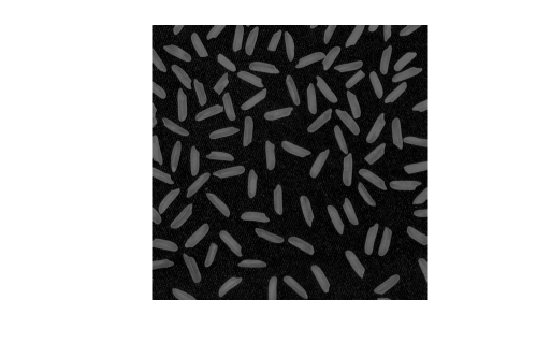Documentation

### This is machine translation

Mouseover text to see original. Click the button below to return to the English version of the page.

To view all translated materials including this page, select Country from the country navigator on the bottom of this page.

# imsubtract

Subtract one image from another or subtract constant from image

## Syntax

``Z = imsubtract(X,Y)``

## Description

example

````Z = imsubtract(X,Y)` subtracts each element in array `Y` from the corresponding element in array `X` and returns the difference in the corresponding element of the output array `Z`.If `X` is an integer array, elements of the output that exceed the range of the integer type are truncated, and fractional values are rounded.```

## Examples

collapse all

This example shows how to subtract two `uint8` arrays. Note that negative results are rounded to 0.

```X = uint8([ 255 0 75; 44 225 100]); Y = uint8([ 50 50 50; 50 50 50 ]); Z = imsubtract(X,Y)```
```Z = 2x3 uint8 matrix 205 0 25 0 175 50 ```

Read a grayscale image into the workspace.

`I = imread('rice.png');`

Estimate the background.

`background = imopen(I,strel('disk',15));`

Subtract the background from the image.

`J = imsubtract(I,background);`

Display the original image and the processed image.

`imshow(I)````figure imshow(J)```Read an image into the workspace.

`I = imread('rice.png');`

Subtract a constant value from the image.

`J = imsubtract(I,50);`

Display the original image and the result.

`imshow(I)````figure imshow(J)```## Input Arguments

collapse all

First array (minuend), specified as a real, nonsparse, numeric or logical array of any dimension.

Second array (subtrahend) to be subtracted from `X`, specified as a real, nonsparse, numeric or logical array. `Y` either has the same size and class as `X`, or `Y` is a scalar of type `double`.

## Output Arguments

collapse all

Difference, returned as a numeric array of the same size as `X`. `Z` is the same class as `X` unless `X` is logical, in which case `Z` is data type `double`. If `X` is an integer array, elements of the output that exceed the range of the integer type are truncated, and fractional values are rounded.